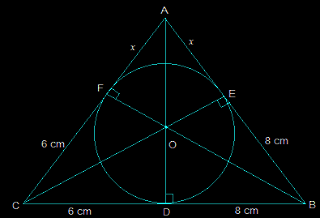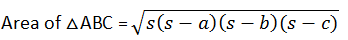Guru

# A triangle ABC is drawn to circumscribe a circle of radius 4 cm such that the segments BD and DC into which BC is divided by the point of contact D are of lengths 8 cm and 6 cm respectively (see Fig. 10.14). Find the sides AB and AC. Q.12

• 1

Please suggest me the best way to solve the problem of question from circles chapter of exercise 10.2 of ncert math of question no.12 how i solve this problem in easy and simple way A triangle ABC is drawn to circumscribe a circle of radius 4 cm such that the segments BD and DC into which BC is divided by the point of contact D are of lengths 8 cm and 6 cm respectively (see Fig. 10.14). Find the sides AB and AC.

Share

1. The figure given is as follows:Consider the triangle ABC,

We know that the length of any two tangents which are drawn from the same point to the circle is equal.

So,

(i) CF = CD = 6 cm

(ii) BE = BD = 8 cm

(iii) AE = AF = x

Now, it can be observed that,

(i) AB = EB+AE = 8+x

(ii) CA = CF+FA = 6+x

(iii) BC = DC+BD = 6+8 = 14

Now the semi perimeter “s” will be calculated as follows

2s = AB+CA+BC

By putting the respective values we get,

2s = 28+2x

s = 14+xBy solving this we get,

= √(14+x)48x ……… (i)

Again, the area of △ABC = 2 × area of (△AOF + △COD + △DOB)

= 2×[(½×OF×AF)+(½×CD×OD)+(½×DB×OD)]

= 2×½(4x+24+32) = 56+4x …………..(ii)

Now from (i) and (ii) we get,

√(14+x)48x = 56+4x

Now, square both the sides,

48x(14+x) = (56+4x)2

48x = [4(14+x)]2/(14+x)

48x = 16(14+x)

48x = 224+16x

32x = 224

x = 7 cm

So, AB = 8+x

i.e. AB = 15 cm

And, CA = x+6 =13 cm.

• 1